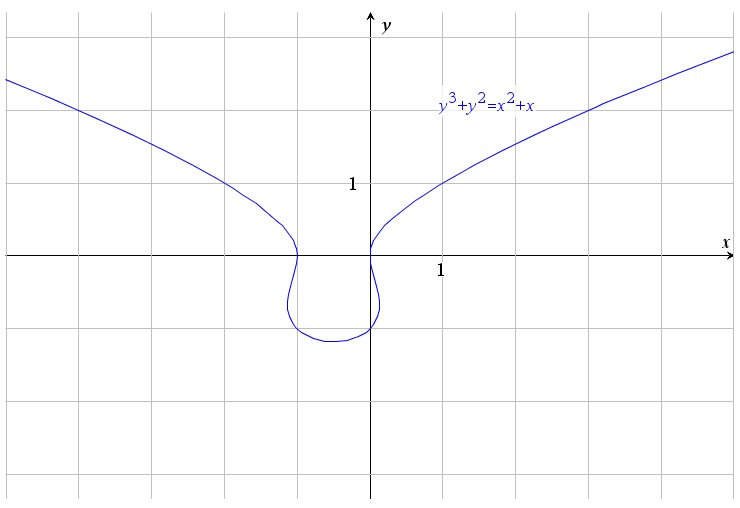# y^3+y^3=x^2+x

Up a level : Differentiation, derivatives
Previous page : The relation y^3+y^2=x^3+x^2
Next page : y^3+y^2=(x^4)y+x^2Let us look at${y^3} + {y^2} = {x^2} + x$

Here we can make x the subject, but just for the sake of it, let’s not do it. Instead we will analyse it in a similar way as the previous example. It will also be a case where we can test our methods without the need for some tool that can do implicit plots. Any calculator will do.

Zeroes

If x is 0 then we get${y^3} + {y^2} = 0$

And thus that y=0 or y=−1.

For y=0 we get$0 = {x^2} + x$

And thus x=0 or x=−1.

Other obvious solutions

The point (1, 1) is quite obviously on the graph.

Asymptotes

If x goes toward infinity y will go toward infinity, since in both cases x2+x will be positive and y3+y2 will be negative for negative values of y. For large x, is dominated by x2 and y2 is dominated by y3, so we get that the graph tend to${y^3} = {x^2}$

or$y = {\left| x \right|^{2/3}}$

close to 0.  Technically you might not get an asymptote using the trick of discarding term that grows much slower than the dominant term, but you will at least get something that behaves somewhat the same.

If x and y is close to 0 we get that our relation is close to${y^2} = x$

Since y3 and x2 goes toward 0 much faster than y2 and x.  We should thus expect the graph to behave like$y = \pm \sqrt x$

close to the origin.

Symmetries

We have two zeroes at −1 and 1. It can be worthwhile to try to shift the relation 1/2 step in the positive direction to see if we get a symmetric relation, and indeed$\begin{gathered} {y^3} + {y^2} = {(x - \tfrac{1}{2})^2} + (x - \tfrac{1}{2}) = (x - \tfrac{1}{2})(x - \tfrac{1}{2} + 1) \hfill \\ \quad \quad \quad = (x - \tfrac{1}{2})(x + \tfrac{1}{2}) \hfill \\ \end{gathered}$

We can now see that we have a symmetry about the y-axis, and thus around the y=−1/2 for the original relation.  That means that we know that the points (−1,−1) and (−2,1) will be on the graph too.

Points with horizontal tangents

To find these we find y´=dy/dx of the relation using implicit derivatives. We get$3{y^2}y' + 2yy' = 2x + 1$

For y´=0 we get$0 = 2x + 1$

Or x=−1/2, i.e. at the line of symmetry.  It might be easiest to use the symmetric version with x=0.${y^3} + {y^2} = - \tfrac{1}{4}$

This gives us that y is about −1.18.

Points with vertical tangents

We can now find x´=dx/dy using implicit derivatives, or take the reciprocal of y´. We have$y'(3{y^2} + 2y) = 2x + 1$

or$y' = \frac{{2x + 1}}{{3{y^2} + 2y}} = \frac{1}{{x'}}$

So to find points where x´=0 we can find where$3{y^2} + 2y = 0$

I.e. at y=0 and y=−2/3

For y=0 we already have that x=−1 or x=0, so we have a vertical slope at those two points.

For y=−2/3 we get${\left( { - \frac{2}{3}} \right)^3} + {\left( { - \frac{2}{3}} \right)^2} = {x^2} + x = \frac{4}{{27}}$

This gives, using an ordinary calculator x=0.13 and x=−1.13 (rounded to two decimals).

Slopes

We can use$y' = \frac{{2x + 1}}{{3{y^2} + 2y}}$

To find the slope at the other points we have found, in particular (0,-1) and (1,1)

For (0,−1) we get y´=1 and thus, by symmetry, −1 at (−1,−1).

For (1,1) we get y’´=3/5 and thus, by symmetry, −3/5 at (−2,1).

Putin it all together

So let us see what we have:It seems rather obvious how to connect these, and indeed we have the graph:Up a level : Differentiation, derivatives
Previous page : The relation y^3+y^2=x^3+x^2
Next page : y^3+y^2=(x^4)y+x^2Last modified: Apr 26, 2019 @ 07:01